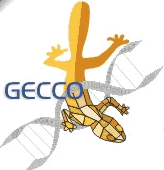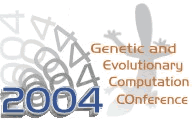June 26 - 30, 2004
Saturday to Wednesday
Seattle, Washington, USA

 Session: MOD - Modularity, regularity and hierarchy in open-ended evolutionary computation Title: A Note on Building-block Identification by Simultaneity Matrix Authors: Chatchawit AporntewanPrabhas Chongstitvatana Abstract: This paper presents a line of research in genetic algorithms (GAs), called building-block identification. The building blocks (BBs) are common structures inferred from a set of solutions. In simple GA, crossover operator plays an important role in mixing BBs. However, the crossover probably disrupts the BBs because the cut point is chosen at random. Therefore, the BBs need to be identified explicitly so that the solutions are efficiently mixed. We propose an approach for identifying BBs. The approach is based on an observation of the compact GA. We observe that the vector elements that correspond to the bits in the same BB are simultaneously updated with a high probability. We formulate the observation to the simultaneity matrix -- an LxL matrix of numbers constructed from a set of L-bit solutions. The matrix element in row i and column j is denoted by mij. The larger the mij is, the higher the dependency is between bit i and bit j. If mij is high, bit i and bit j should be passed together to prevent BB disruption. Our approach is validated for additively decomposable functions (ADFs) and hierarchically decomposable functions (HDFs). In terms of scalability, our approach shows a polynomial relationship between the number of function evaluations required to reach the optimum and the problem size. A comparison between the simultaneity matrix and the hierarchical Bayesian optimization algorithm (hBOA) shows that the matrix computation is 10 times faster and uses 10 times less memory than constructing the Bayesian network.